##Quadratically Speaking

Much of what is going on in our (idealized) Universe is quadratic in nature in one way or another. In part this is due to the fact that moving bodies subjected to the laws of Gravity follow quadratic projectiles. Mathematically speaking the (x,y) coordinates of a freely moving body are related to each other as y = ax2 + bx + c, where a,b, and c are constants. The sign of a and b depends on the directions of the coordinate system used. In the case of the usual system (y is moving upward, x is moving to the right), a is negative and b is positive.

Not surprising therefore, quadratic functions are used extensively in animation, and in this sense Walt Disney Studios are very much quadratically oriented.

Such applications provide excellent teaching material.

Here I present one example that I have been using for many years. The exposition is designed for lecturers/tutors, rather than students, but I suspect that good students should have no problem digesting the material on their own.

The example in question is a real world application of quadratic functions and has to do with a body, say a young kangaroo called Kangee, trying to clear a hurdle, say a small bush called Bushy, by hopping over it. To animate this situation we let the (x,y) coordinates of Kangee be time (t) dependent. So (x(t),y(t)) is the position of Kangee at time t in the assumed x-y plane. We know (from basic physics) that y(t) = x2(t) + bx(t) + c, where the parameters a,b, and c depends on Kangee's horizontal and vertical velocities at the time of lift-off and of course ... the assumed gravitational force. Here and elsewhere in this discussion we assume that the objective is to determine a projectile that is as tight as possible to the obstacle.

Figure 1(a) depicts the projectile of a small red ball thrown in some upward/right direction. The ball is shown in three positions. Figure 1(b) shows how this projectile can be used to describe how the ball clears a triangular obstacle.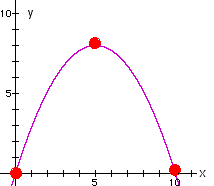(a)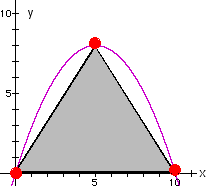(b)
Figure 1

To determine the values of the parameters a,b, and c of the projectile we solve a system of three equations associated with these three unkowns, utilizing the coordinates of the three vertices of the obstacle.

 (x,y) = (0,0): 0 = a*0 + b*0 + c >> 0 = 0 + 0 + c (x,y) = (0,10): 0 = a(102) + 10b + c >> 0 = 100a + 10b + c (x,y) = (5,8): 8 = a(52) + 5b + c >> 8 = 25a + 5b + c

The solution is a=-0.32, b=3.2, c=0, so the quadratic (x,y) relationship is y = -0.32x2 + 3.2x.

More generally, for an equal-sided triangle with height = H and width = W we have,

 (x,y) = (0,0): 0 = a*0 + b*0 + c (x,y) = (0,W): 0 = a*W2 + b*W + c (x,y) = (W/2,H): H = a*(W/2)2 + b* (W/2)+ c

The solution is

a = - 4H/W2 ; b = 4H/W , c = 0

so the quadratic (x,y) relationship is

y = -(4H/W2)x2 + (4H/W)x.

Note that if x(0) = y(0) = 0, then c = 0 and if in addition y(T)=0 for some t = T != 0, then b = -a*x(T) and the quadratic (x,y) relationship is as follows: y(t) = a*(x2(t) - x(T)x(t)).

Now there is a minor complication with the above idealized case: the ball is assumed to be of zero width!!! If the moving body is relatively wide, its width must be incorporated into the analysis, for otherwise the animation will not be very effective: the moving body will not clear the obstacle. This practical issue is illustrated in Figure 2.Figure 2

To fix this minor bug we have to do two things: first we have to increase the width of the obstacle. If we let D denote the width of the moving body, then we increase the width of the obstacle from W to W+D. This is simple.

But ... we also have to increase the height of the obstacle, for otherwise the moving body will not clear the obstacle. This is illustrated in Figure 3(a).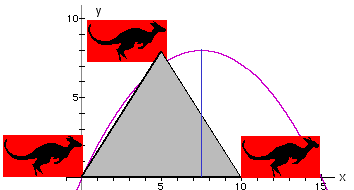(a)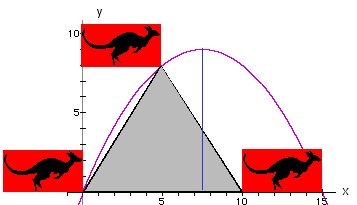(b)
Figure 3

Note that the moving body (the red rectangle) on the upper part of Figure 3(a) does not clear the obstacle.

The question therefore arises: what should be the new (increased) height of the obstacle so that the moving body will clear the original obstacle? This magical height is used in Figure 3(b) to display the desired effect.

It is not necessary to determine the value of the proper new height directly in the sense that this value will be determined by a proper choice of the parameters a, b and c. The clue as to how the values of these parameters should be computed is given in Figure 3(b): we simple have to make sure that the projectile passes through the three points (x,y) = (0,0), (x,y) = (W+D,0) and (x,y) = (W/2, H), recalling that W and H denote the width and height of the original obstacle, respectively. Thus, we have to solve the following system:

 (x,y) = (0,0): 0 = a*0 + b*0 + c (x,y) = (0,W+D): 0 = a*(W+D)2 + b*(W+D) + c (x,y) = (W/2,H): H = a*(W/2)2 + b*(W/2)+ c

The solution is:

a = -4H/(W2+2D*W), b = 4H*(W+D)/((W2+2D*W)) , c = 0

hence the quadratic relationships is as follows:

y = -(4H/(W2+2D*W))*x2 + -(4H*(W+D)/(W2+2D*W))x

Thus, for the case where H=10 and D=5, we have

y = -0.16x2 + 2.4x

This implies that the highest point on the projectile is (x,y)=(7.5,9), observing that for x=(W+D)/2 we have y=9.

Comments:

1. The above analysis is for the case where the obstacle is an equal-sided triangle standing on its other side. It would be good for students to think about the complexities involved in dealing with obstacles having other shapes, eg. Circle, square.
2. By the same token, the above analysis is based on the assumption that the moving body is a rectangle. What happens if the moving body is of a different shape, say a ball, or a kangaroo?
3. More advanced students may wish to consider the question of how one incorporates the value of the gravitational coefficient (g) in an animation of the motion of the body.

It is time now to practice what we preach!

First we provide you with a very light and extremely naive facility for drawing quadratic functions of the type we discussed above.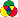More excitedly, we invite you to our on line Q-Lab to experiment with some of the ideas we discussed above.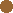Buddy Hurdle Gravity Kitty the catRabbit 1Rabbit 2ElephantBugTurtleKangarooAlligatorRooster Dog signCactusCarTree 1Tree 2 12345678910 Wecome to the lab! It will be ready shortly.

Disclaimer: This page, its contents and style, are the responsibility of the author (Moshe Sniedovich) and do not represent the views, policies or opinions of The University of Melbourne.

Disclaimer: This page, its contents and style, are the responsibility of the author (Moshe Sniedovich) and do not represent the views, policies or opinions of the organizations he is associated/affiliated with.